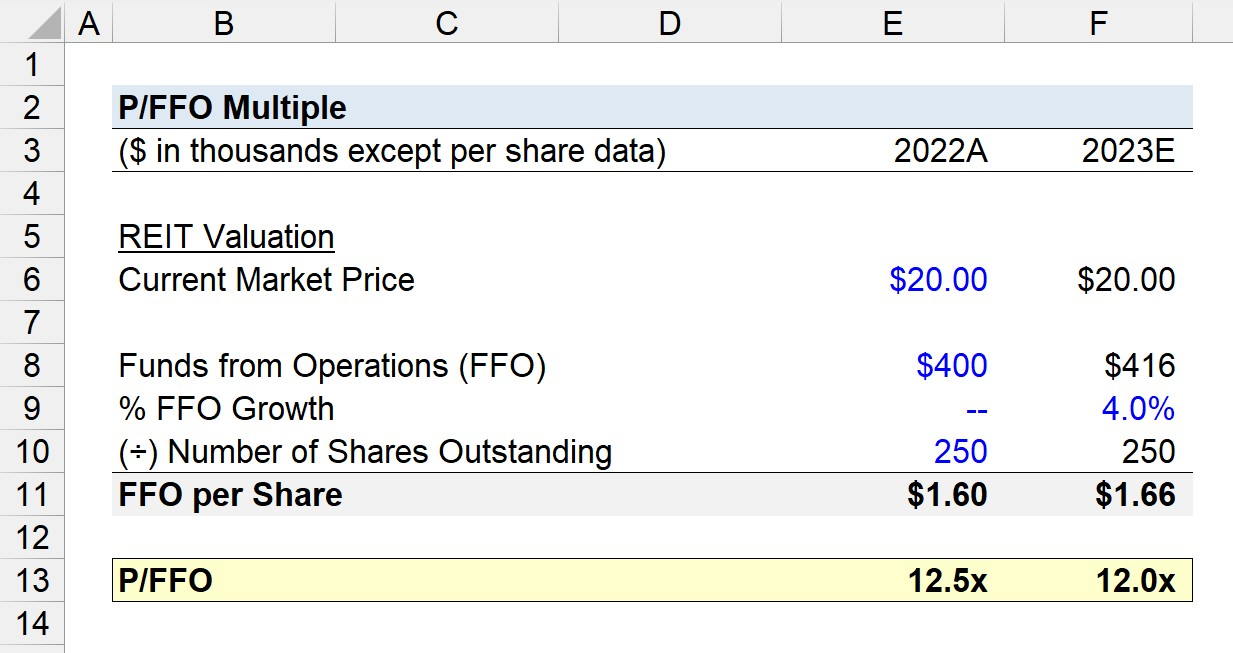Welcome to Wall Street Prep! Use code at checkout for 15% off.# P/FFO Multiple

Guide to Understanding the P/FFO Multiple (Price to FFO Ratio)## How to Calculate P/FFO?

One method to value real estate investment trusts (REITs) is by using multiples, such as the P/FFO multiple.

The P/FFO multiple is the ratio between the current market price of a REIT and its funds from operations (FFO).

• Price → The “Price” component of the P/FFO ratio refers to the current market price, or share price, of the REIT as of the latest closing date.
• FFO → The “FFO” component is the funds from operations (FFO) of the REIT, expressed on a per-share basis so that the numerator and denominator align.

The market price of a REIT is readily available via online resources like Bloomberg, whereas the FFO must be manually calculated.

FFO stands for “Funds from Operations” and is a non-GAAP financial measure widely recognized in the REIT market.

In practice, FFO is a standardized metric used to analyze the operating performance of REITs  – designed by Nareit – because traditional GAAP metrics like net income fall short of reflecting the true performance of a REIT.

Hence, FFO is instead used by most practitioners because the metric is more reliable in portraying the “actual” performance of REITs.

While REITs report their FFO in the supplementary sections of their financial reports and non-GAAP filings (e.g. investor presentations), the recommended approach is to arrive at FFO using your own calculations.

Why? Calculating the FFO of a REIT manually – instead of relying on the figures provided by the REIT’s management team – leads to a better understanding of the REIT’s operating performance and the adjustments applied to the metric.

In order to calculate the funds from operations (FFO) metric, net income is reconciled starting with the add-back of the non-cash depreciation expense of real estate assets.

Once the depreciation is added back to net income, other adjustments include subtracting the gain on asset sales and adding back one-time items, such as asset impairments.## P/FFO Formula

The formula to calculate the P/FFO multiple is the ratio between the market price and funds from operations (FFO) on a per-share basis.

P/FFO = Current Market Price ÷ FFO per Share

The FFO per Share metric can be determined using the following formula.

FFO per Share = Funds from Operations (FFO) ÷ Number of Shares Outstanding

The FFO input can be the current funds from operations (FFO) of the REIT – the FFO in the trailing twelve months (“TTM”) – or on an estimated, forward-basis (i.e. next twelve months, “NTM”).

## P/FFO Calculator

We’ll now move to a modeling exercise, which you can access by filling out the form below.#### Excel TemplateSubmitting...

## REIT P/FFO Calculation Example

Suppose in the fiscal year ending 2022, a publicly-traded REIT reportedly generated \$400k in funds from operations (FFO) per its non-GAAP filings.

Currently, the REIT’s market price is \$20.00 per share. In the next period, the REIT’s FFO is expected to grow by 4.0% YoY, while the number of shares outstanding will remain constant.

• Current Market Price = \$20.00
• Funds from Operations (FFO) – 2022A = \$400k
• % FFO Growth = 4.0% YoY
• Funds from Operations (FFO) – 2023E = \$400k × (1 + 4.0%) = \$416k

If the REIT has a total of 250k shares (or units) in circulation, what is the P/FFO of the REIT on a trailing and forward basis?

• Number of Shares Outstanding = 250k
• FFO per Share – 2022A = \$400k ÷ 250k = \$1.60
• FFO per Share – 2023E = \$416k ÷ 250k = \$1.66

The P/FFO of the REIT can be determined by dividing the current market price by the corresponding FFO per share in 2022A (TTM) and 2023E (NTM).

• P/FFO Multiple – 2022A (TTM) = \$20.00 ÷ \$1.60 = 12.5x
• P/FFO – 2023E (NTM) = \$20.00 ÷ \$1.66 = 12.0x

In conclusion, the REIT is currently trading at a P/FFO multiple of 12.5x based on its most recent earnings (TTM) and 12.0x on a forward basis (NTM).20+ Hours of Online Video Training

### Master Real Estate Financial Modeling

This program breaks down everything you need to build and interpret real estate finance models. Used at the world's leading real estate private equity firms and academic institutions.

Inline Feedbacks